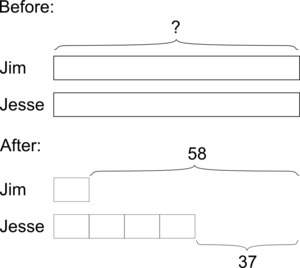# Jim and Jesse's Money

Alignments to Content Standards: 6.RP.A.3

Jim and Jesse each had the same amount of money. Jim spent $\$58$to fill the car up with gas for a road-trip. Jesse spent$\$37$ buying snacks for the trip. Afterward, the ratio of Jimâ€™s money to Jesseâ€™s money is $1:4$. How much money did each have at first?

## Solutions

Solution: Use two bar diagramsWe know that Jesse has \$21 more than Jim after they make their purchases, because Jim spent \$21 more than Jesse. Since the ratio of money they have remaining is $1:4$, we know this difference is 3 times what Jim has left.

$3 \text{ units } = \$58 - \$37 = \$211 \text{ unit } = \$7$

We should add what Jim has left to what he spent:

$\$58 + \$7 = \$65$They each had$\$65$ at first.

Solution: Same story, slightly different analysisWe know that Jim spent \$21 more than Jesse, so Jim has \$21 less than Jesse after they make their purchases. Since Jim has only $\frac14$ as much money as Jesse has, the difference between the two amounts is $\frac34$ of the amount of money Jesse has. So 21 is $\frac34$ of the amount of money Jesse has. Therefore, $\frac14$of the amount of money Jesse has is one third of this, or 7. So Jesse has $4 \cdot 7=28$; and since he spent 37, he originally had $28 + 37=65$. So Jim and Jesse each originally had \\$65.